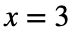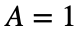# Problem 57512. Easy Sequences 89: Double Summation of a Trigonometric Product

Given and angle A in radians and a positive integer x, evaluate the following product summation:which 'directly' translates to Matlab as:
>> PS = @(A,x) sum(arrayfun(@(n) sum(arrayfun(@(m) prod(arrayfun(@(k) 2*sin(k*pi/m+A),0:m-1)),1:n)),1:x));
For exampleand:
>> x = 3; A = 1;
>> PS(A,x)
ans =
8.9683

### Solution Stats

100.0% Correct | 0.0% Incorrect
Last Solution submitted on Jan 10, 2023

### Community Treasure Hunt

Find the treasures in MATLAB Central and discover how the community can help you!

Start Hunting!